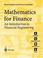### Financial Mathematics

#### Course Description

The course introduces the concepts of both compounded and continuosly compounded interest, risk-free assets, term structure of interest rates and fixed income securities. The basics from stochastic calculus as a necessary instrument for quantitative analysis of financial markets, investment strategies, the concept of arbitrage and the funadamental theorem of finance are provided. Optimality in the sense of maximazing the rate of return, minimizing the variance in the rate of return and minimizing risk to the investor are explored. Effects of correlated movements in the values of investments are modelled and the Capital Asset Pricing Model (CAPM) is introduced. Models describing the price dynamics in both discrete time as the binomial model and continuous time as the model leading to the Black-Scholes formula are analyzed. The basics from option pricing and hedging are introduced.

#### General Competencies

Fundamental knowledge in the field of modern financial mathematics and quantitative finance modelling. Understanding the theoretical background necessary for the quantitative analysis of financial markets under conditions of uncertainty.

#### Learning Outcomes

1. recognize the basis of financial modelling with an emphasis on applied finance
2. explain stochastic calculus as a basic tool for research in modern financial mathematics
3. apply basics of probability and statistics for the analysis of financial instruments in practice
4. analyze testing of financial hypotheses using statistical tests
5. develop theoretical basics necessary for the quantitative analysis of financial markets
6. evaluate financial assets under conditions of uncertainty

#### Forms of Teaching

Lectures

Lectures are organized through two cycles. The first cycle consists of 7 weeks of classes and mid-term exam, a second cycle of 6 weeks of classes and final exam. Classes are conducted through a total of 15 weeks with a weekly load of 3 hours.

Exams

Mid-term exam. Final exam.

Consultations

Consultations are organited every week.

Seminars

Seminars

#### Grading Method

Continuous Assessment Exam
Type Threshold Percent of Grade Threshold Percent of Grade
Attendance 0 % 5 % 0 % 5 %
Mid Term Exam: Written 0 % 45 % 0 %
Final Exam: Written 0 % 50 %
Exam: Written 0 % 95 %

#### Week by Week Schedule

1. Simple and Compound Interest. Continuosly Compounded Interest. Present Value. Annuities. Amortization of a Debt.
2. Comparison of Investment projects. Internal rate of return. Capital Budgeting.
3. Term Structure of Interest Rates. Fixed Income Instruments.
4. Zero-Coupon Bonds. Coupon Bonds. Nominal value. Maturity.
5. Variable Interest Rates. Duration. Modified Duration. Portfolio of Bonds. Dynamic Hedging.
6. General Term Structure. Forward rates.
7. Random Walks. Brownian Motion. An Intuitive Idea of a Stochastic Process.
8. Mid-term exam
9. Stock Market Example. Lognormal Distribution and Lognormal Random Variables.
10. Investment Strategies. The Concept of Arbitrage. Fundamental Theorem of Asset Pricing.
11. Discrete Time Market Models. One period Financial Market Model. The Binomial Model.
12. Risk-neutral probabilities. Lack of Arbitrage. Martingale property.
13. Continuous Time Market Models. Investment Strategies. European Options. Risk-neutral Valuation.
14. Black-Scholes Formula. Black-Scholes Partial Differential Equation. Market Price of Risk. Itô's Lemma.
15. Final exam.

#### Study Programmes

##### University graduate
Computer Engineering (profile)
Mathematics and Science (2. semester)
Computer Science (profile)
Mathematics and Science (2. semester)
Control Engineering and Automation (profile)
Mathematics and Science (2. semester)
Electrical Engineering Systems and Technologies (profile)
Mathematics and Science (2. semester)
Electrical Power Engineering (profile)
Mathematics and Science (2. semester)
Electronic and Computer Engineering (profile)
Mathematics and Science (2. semester)
Electronics (profile)
Mathematics and Science (2. semester)
Information Processing (profile)
Mathematics and Science (2. semester)
Software Engineering and Information Systems (profile)
Mathematics and Science (2. semester)
Telecommunication and Informatics (profile)
Mathematics and Science (2. semester)
Wireless Technologies (profile)
Mathematics and Science (2. semester)

#### LiteratureM. Capiński and T. Zastawniak (2003.), Mathematics for Finance: An Introduction to Financial Engineering, Springer-Verlag
John C. Hull (2005.), Options, Futures and Other Derivatives (6th Edition), Prentice-Hall
S. R. Pliska (1997.), Introduction to Mathematical Finance: Discrete-Time Models, Blackwell Publishers

#### General

ID 68986
Summer semester
4 ECTS
L1 English Level
L1 e-Learning
45 Lectures
0 Exercises
0 Laboratory exercises
0 Project laboratory

85 Excellent
75 Very Good
60 Good
50 Acceptable